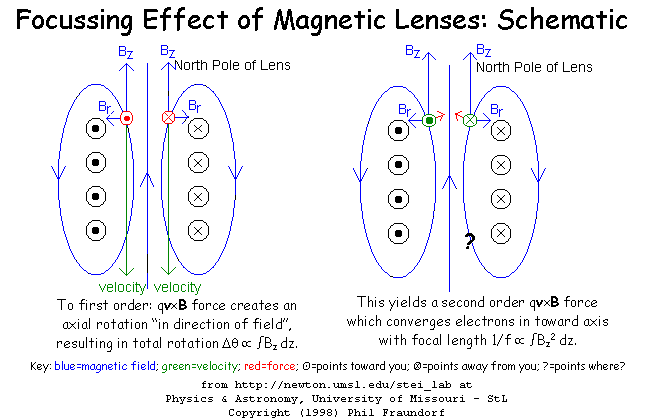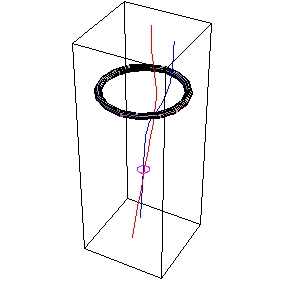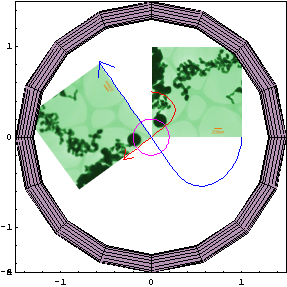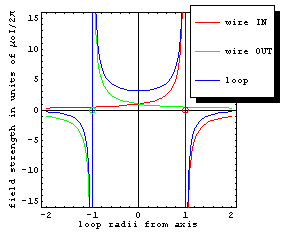# The Solenoid/MagneticLens Field

Here the magnetic field of a two-turn solenoid is calculated* on a set of cartesian grid points located at the base of each plotted arrow. As you can see, a cluster of near-parallel magnetic field lines is created when a current runs through the coil. You can reorient the model by dragging the mouse over it for a clearer look at its three-dimensional structure. Puzzler #1: Which way is the current in this solenoid flowing, i.e. is current moving in the direction of the larger magnetic field arrows, or vice versa?

* (by integrating Biot-Savart's dBoIds×r/r3 over the wire's length)

The solenoid design above underlies the concept of inductive circuit elements in AC circuits. By arranging it so that changing magnetic flux (generated by currents in the wire) induce Lenz-law (change-avoiding) voltages** in that very same wire, these elements play a key role in tuning electromagnetic broadcasts. Moreover, linkage of two such coils in transformers is key to making AC power helpful for electric power distribution, as well as for low voltage battery rechargers.

** (namely VL=-L dI/dt, where for an N-turn solenoid of radius r, inductance L=NΦB/I~Nμoπr/2)

One can also think of the solenoid above as a convergent lens for charged particles, as illustrated in the diagram below. This was the basis for invention of the electron microscope in the first half of the 20th century. It turns out one cannot similarly build divergent electron lenses, even though these are quite useful e.g in light microscopes for correcting spherical aberration. This has kept the resolution of electron microscopes from moving much below the spacing between atoms in solids (i.e. around 2 Ångstroms) until emergence (in the current decade) of dynamic aberration-corrections that let multi-million dollar electron microscopes at last move into the sub-Ångstrom regime. Eventually, it is hoped that atoms in images will begin to look (thanks to scattering from their nuclei) like stars in the night sky. Puzzler #2: What direction is the force, on an electron with azimuthal velocity into the screen, at the question mark in the image below?Below, check out the trajectories*** of two different electrons (red and blue curves) from the point of view of their initial direction of travel down the lens axis. As axis-parallel electrons arrive at the lens, the qv×B Lorentz force causes off-axis electrons (which see a radial B-component) to begin an azimuthal spin (here clockwise) around the lens axis. This rotates the image a finite amount (proportional to lens current) by the time the electrons leave the lens. As a result, electron lenses naturally rotate the images that travel down their column.

*** (obtained by integrating the force equation mx''=qx'×B[x] over time from a given starting point and velocity)The resulting azimuthal velocity in turn results in a radially-inward focusing force (proportional to lens current squared) that converges all rays into a focal point beneath the lens, from there to proceed in an ever widening pattern outward (arrow heads) until the next lens, aperture, or detector is encountered. This is how the objective lens of an electron microscope works, some of which are able to produce high quality images with final magnifications over 100 million times. Unlike glass lenses, it's possible to change an electron lens' focal length simply by changing the current through its windings. Although trajectory simulations like that above are needed for precise lens design, magnetic lenses can thus be approximated with the familiar equation for a thin convex light lens****, along with an equation for image magnification and rotation angle. Puzzler #3: Converging glass lenses result in an up-down/left-right inversion as the rays pass through the focal point. Is that similarly true of the magnetic lens above?

**** (namely 1/i + 1/o = 1/f, where focal length f is inversely proportional to lens current squared.)

Contrast in electron images sometimes looks familiar, but it usually arises from very different mechanisms than those we are familiar with from light images. One example of this is the deBroglie phase contrast oft responsible for lattice fringes in high resolution electron images. And then there is the back-focal plane of the lens i.e. the horizontal plane (marked with a magenta oval above) in which initially parallel electron paths converge to a point on the lens axis. It's the home of the specimen's electron diffraction pattern. This is a map of electron intensity leaving the specimen as a function of scattering angle, rather than as a function of lateral postion on the specimen. In the image below, an objective aperture in that back-focal plane is being used to only let those electrons through that get focused onto the optic axis. For more on reciprocal structures which "live" in this back-focal plane, follow this link here.Speaking of electron optics, this short movie provides a millihuman tour of the electron microscope column pictured above. The trek begins in the electron gun's Wehnelt just beneath the pointed LaB6 emitter source, and ends on the image viewing screen at the bottom of the column. One clue to the kinds of detective work made possible by electron optics is provided here.

• What tools and strategies would you use to carry out the calculations illustrated above?
• Here are links to similarly interactive models of the electric field associated with charge on a capacitor and voltage across a resistor.
• Here are links to real time trajectory simulators for a current loop, and a gravitational/electrostatic field.
Our goal here is to provide quantitative "vehicle steering challenges", through various fields of force.
• Other recent electricity and magnetism visual puzzlers may be found here.

This site is hosted by the Department of Physics and Astronomy (and Center for Molecular Electronics) at UM-StL. The person responsible for mistakes and copyright (2005-6) is P. Fraundorf. MindQuilts site page requests are on their way up (we think) to about a million per year. Requests for a "stat-counter linked subset of pages" since 4/7/2005: .